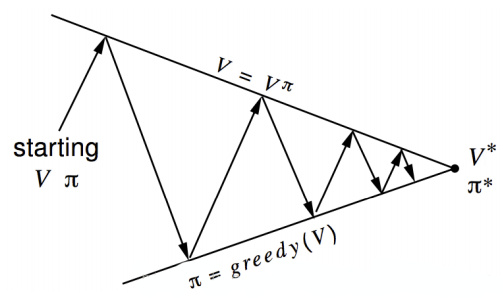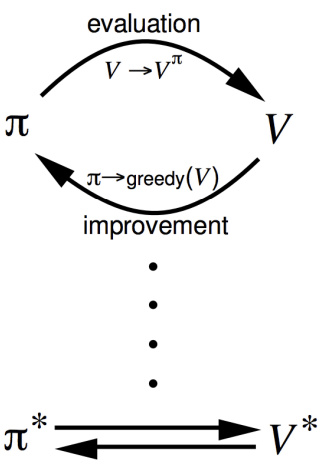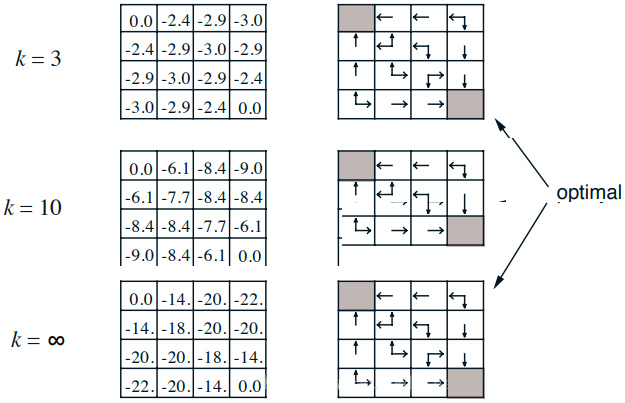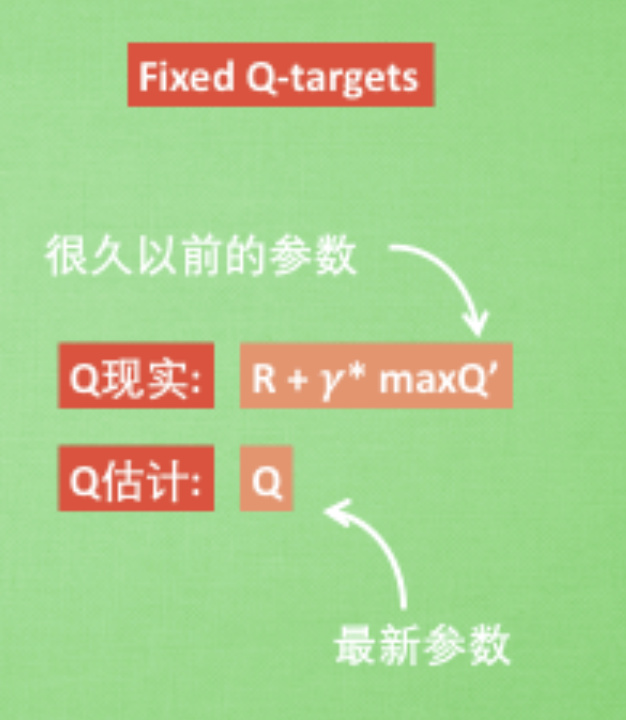## 1. 前言

• 动作 Action
• 观察 Observation
• 反馈值 Reward

$$a=\pi(s)$$

$$\pi(a|s)$$

$$\{s_1,a_1,r_1,s_2,a_2,r_2,…s_t,a_t,r_t\}$$

## 2. 马尔科夫决策过程

MDP只需要用一句话就可以说明白，就是 “未来只取决于当前”，专业点说就是下一步的状态只取决于当前的状态，与过去的状态没有关系。

$$P(s_{t+1}|s_t)=P(s_{t+1}|s_t,s_{t-1},…s_1,s_0)$$

P为概率。简单的说就是下一个状态仅取决于当前的状态和当前的动作。

• $$S$$ ：所有可能状态的集合， $$s \in S$$，$$s$$ 表示某个特定状态
• $$A$$ ：针对每个状态，我们都要做出动作，这些动作的集合就是 $$A$$； $$a \in A$$，有限动作 action 集合， $$a$$ 表示某个特定动作
• $$P_{sa}$$ ：状态转换分布（statetransition distribution），如果我们在状态 $$s$$ 中采取了动作 $$a$$ ，系统会转移到一个新的状态，状态转换分布描述了转移到哪个状态的概率分布。
• $$R$$ ：回馈函数（rewardfunction），增强学习的核心概念，描述了动作能够产生的回报。比如 $$R_π(s,a)$$ 描述了在状态 $$s$$ 下采用策略 $$\pi$$ 所对应的动作 $$a$$ 的回报，也叫做立即回报，回馈函数可以有不同的表达形式。
• $$\pi(s)\rightarrow a$$： 策略 policy，根据当前 state 来产生 action，可表现为 $$a=\pi(s)$$ 或 $$\pi(a|s) = P[a|s]$$，后者表示某种状态下执行某个动作的概率

## 3. 价值函数

$$G_t = R_{t+1} + \lambda R_{t+2} + … = \sum_{k=0}^\infty\lambda^kR_{t+k+1}$$

• R 是 Reward 反馈
• $$λ$$ 是 discount factor 折扣因子，一般小于 1，就是说一般当下的反馈是比较重要的，时间越久，影响越小。

• 直接优化策略 $$\pi(a|s)$$ 或者 $$a = \pi(s)$$ 使得回报更高
• 通过估计 value function 来间接获得优化的策略。道理很简单，既然我知道每一种状态的优劣，那么我就知道我应该怎么选择了，而这种选择就是我们想要的策略。

1. 状态价值函数 $$V$$ ：从状态 $$x_0$$ 开始, 所有的动作 $$a$$ ，都是执行某个策略 $$π$$ 的结果，最后求每个动作带来累积奖赏
2. 动作价值函数 $$Q$$ ：从状态 $$x_0$$ 开始，先执行动作 $$a_0$$ ， 然后再执行某个策略 $$π$$ ，再求相应的积累奖赏

### 3.1 State-Value function 状态价值函数

$$v(s) = \mathbb E[G_t|S_t = s]$$

$$V^{\pi}(s) =\mathbb E_{\pi}[ R(s_0, a_0) + γR(s_1, a_1)+ γ^2R(s_2, a_2) + … | s_0= s ]$$

### 3.2 Action-Value function 动作价值函数

$$Q^{\pi}(s,a) = \mathbb E[R_{t+1}+\lambda R_{t+2} + \lambda ^2R_{t+3} + …|S_t = s,A_t=a]$$

\begin{align} Q^\pi(s,a) & = \mathbb E[r_{t+1} + \lambda r_{t+2} + \lambda^2r_{t+3} + … |s,a] \\ & = \mathbb E_{s^\prime}[r+\lambda Q^\pi(s^\prime,a^\prime)|s,a] \end{align}

## 4. Bellman 方程

$$P^a_{ss\prime} = P(S_{t+1}=s\prime|S_t =s, A_t =a)$$

$$G_t = R_{t+1} + \lambda R_{t+2} + … = \sum_{k=0}^\infty\lambda^kR_{t+k+1}$$

$$v(s) = \mathbb E[G_t|S_t = s]$$

$$v_{\pi}=\sum_{a\in A}P(a|s)\left(R^a_s+\lambda\sum_{s\prime \in S}P^a_{ss\prime}v_{\pi}(s\prime)\right)$$

\begin{align} v(s) & = \mathbb E[G_t|S_t = s] \\ & = \mathbb E[R_{t+1}+\lambda R_{t+2} + \lambda ^2R_{t+3} + …|S_t = s] \\ & = \mathbb E[R_{t+1}+\lambda (R_{t+2} + \lambda R_{t+3} + …)|S_t = s] \\ & = \mathbb E[R_{t+1} + \lambda G_{t+1}|S_t = s] \\ & = \mathbb E[R_{t+1} + \lambda v(S_{t+1})|S_t = s] \end{align}

$$v(s) = \mathbb E[R_{t+1} + \lambda v(S_{t+1})|S_t = s]$$

$$v_{\pi}(s) = \mathbb E[R_{t+1} + \lambda v_{\pi}(S_{t+1})|S_t = s]$$

$$q_{\pi}(s,a) = \mathbb E_{\pi}[R_{t+1} +\lambda q_\pi(S_{t+1},A_{t+1})|S_t =s,A_t = a]$$

## 5. 最优化

### 动态规划

• 最优子结构：最优解法能被分解到多个子问题中
• 重叠子问题：子问题能重复多次且解法能被重复利用、

MDP需要解决的问题有两种：

• 第一种是 prediction，它已知MDP的 $$S,A,P,R,γ$$ 以及 policy，目标是算出在每个状态下的 value function(值函数其实就是问题的目标，一般都是跟 reward 有关的函数，例如 Atari 小游戏，一般值函数就是累计的得分的期望。目标一般就是最大化这个值函数。
• 而第二种是control，它已知 MDP 的 $$S,A,P,R,γ$$ 但是 policy 未知（即动作 $$a_t$$ 未知），因此它的目标不仅是计算出最优的 value function 而且要给出最优的 Policy。

### 5.1 Optimal value function 最优价值函数

$$V^*(s,a) = \max_\pi V^\pi(s,a)$$

$$Q^*(s,a) = \max_\pi Q^\pi(s,a)$$

$$Q^*(s,a) = \mathbb E_{s^\prime}[r+\lambda \max _{a^\prime}Q^*(s^\prime,a^\prime)|s,a]$$

![](/img/2018-08-05-RL-1.jpg) ![](/img/2018-08-05-RL-2.jpg)

### 5.2 Policy Iteration 策略迭代

• Policy evaluation ：根据每一次的给出策略估计 $$v_π$$
• Policy improvement：根据 Greedy poilcy 和之前得到的 $$v_π$$ 获得当前策略 $$π′$$

Policy Iteration的目的是通过迭代计算value function 价值函数的方式来使policy收敛到最优。Policy Iteration 本质上就是直接使用 Bellman 方程而得到的：

![](/img/2018-08-05-RL-3.jpg)

### 5.3 Value Iteration 价值迭代

Value Iteration 则是使用 Bellman 最优方程得到：

![](/img/2018-08-05-RL-4.jpg)

![](/img/2018-08-05-RL-5.jpg)

![](/img/2018-08-05-RL-6.jpg)

## 6. Q-Value (Quality-Value)

Q Learning的思想完全根据value iteration得到。但要明确一点是value iteration每次都对所有的Q值更新一遍，也就是所有的状态和动作。但事实上在实际情况下我们没办法遍历所有的状态，还有所有的动作，我们只能得到有限的系列样本。因此，只能使用有限的样本进行操作。那么，怎么处理？Q Learning提出了一种更新Q值的办法：

$$Q(S_{t},A_{t}) \leftarrow Q(S_{t},A_{t})+\alpha({R_{t+1}+\lambda \max _aQ(S_{t+1},a)} - Q(S_t,A_t))$$

![](/img/2018-08-05-RL-7.jpg)

![](/img/2018-08-05-RL-8.jpg)

$$\text{update = learing_rate * (q_target - q_predict)}$$

$$\text{学习率 * (真实值 - 预测值)}$$

## 7. Exploration and Exploitation 探索与利用

• 随机的生成一个动作
• 根据当前的Q值计算出一个最优的动作，这个 policy $$\pi$$ 称之为 greedy policy 贪婪策略。也就是 $$\pi(S_{t+1}) = arg\max _aQ(S_{t+1},a)$$

INITIAL_EPSILON = 0.5 # starting value of epsilon
FINAL_EPSILON = 0.01 # final value of epsilon

## 8. 详解Q-Learning

### 8.1 Value Function Approximation 价值函数近似

$$Q(s,a) = f(s,a)$$

f可以是任意类型的函数，比如线性函数：

$$Q(s,a) = w_1s + w_2a + b$$

$$Q(s,a) = f(s,a,w)$$

$$Q(s,a)\approx f(s,a,w)$$

### 8.2 Q值神经网络化！

$$Q(S_{t},A_{t}) \leftarrow Q(S_{t},A_{t})+\alpha({R_{t+1}+\lambda \max _aQ(S_{t+1},a)} - Q(S_t,A_t))$$

Q值的更新依靠什么？依靠的是利用 Reward 和 Q 计算出来的目标Q值：

$$\text{Target-Q : }\ \ R_{t+1}+\lambda \max _aQ(S_{t+1},a)$$

![](/img/2018-08-05-RL-9.jpg)
\$$s^\,a^\$$ 即下一个状态和动作

**前边提到**，每一组 (state, action, reward, state_) 为一次序列：
• state (observation) 为目前状态，传递给 q-eval net 得到预计值 (即输入状态值, 输出所有的动作值)；
• state_ 为下一步状态，传递给 q-target net 得到目标值，之后可以得到 $$\max _aQ(S_{t+1},a)$$ ，在之后得到 $$R_{t+1}+\lambda \max _aQ(S_{t+1},a)$$

![](/img/2018-08-05-RL-10.jpg)

![](/img/2018-08-05-RL-11.jpg)

![](/img/2018-08-05-RL-12.jpg)

### 8.3 Experience Replay 经验回放

Q learning 是一种 off-policy 离线学习法, 它能学习当前经历着的, 也能学习过去经历过的, 甚至是学习别人的经历. 所以每次 DQN 更新的时候, 我们都可以随机抽取一些之前的经历进行学习. 随机抽取这种做法打乱了经历之间的相关性, 也使得神经网络更新更有效率。

![](/img/2018-08-05-RL-13.jpg)

Experience Replay 的动机是：

• 深度神经网络作为有监督学习模型，要求数据满足独立同分布，
• 但 Q Learning 算法得到的样本前后是有关系的。为了打破数据之间的关联性，Experience Replay 方法通过存储-采样的方法将这个关联性打破了。

### 8.4 Fixed Q-targets

Fixed Q-targets 也是一种打乱相关性的机理, 如果使用 fixed Q-targets, 我们就会在 DQN 中使用到两个结构完全相同但参数不同的神经网络 (有时差), 预测 Q 估计 的神经网络 (evaluate net) 具备最新的参数, 而预测 Q 现实 的神经网络 (target net) 使用的参数则是很久以前的

target_net 用于预测 q_target 目标值, 他不会及时更新参数.

eval_net 用于预测 q_eval 估计值, 这个神经网络拥有最新的神经网络参数

![](/img/2018-08-05-RL-14.jpg)### 8.5 总结

$$\text{Q(state, action) = Q(state, action) + }\alpha\text{ (R(state, action) + }\gamma \text{ Max[Q(next state, all actions)] - Q(state, action))}$$

$$\text{Q(state, action) = R(state, action) +} \gamma\text{ Max[Q(next state, all actions)]}$$

• state： 表示 Agent 当前状态。
• action： 表示 Agent 在当前状态下要做的行为。
• next state： 表示 Agent 在 state 状态下执行了 action 行为后达到的新的状态。
• Q(state, action)： 表示 Agent 在 state 状态下执行了 action 行为后学习到的经验，也就是经验分数。
• R(state, action)： 表示 Agent 在 state 状态下做 action 动作后得到的即时奖励分数。
• Max[Q(next state, all actions)]： 表示 Agent 在 next state 状态下，自我的经验中，最有价值的行为的经验分数。
• Gamma： $$\gamma$$ ，表示折损率，也就是未来的经验对当前状态执行 action 的重要程度。

**算法流程:**

Agent 通过经验去学习。Agent将会从一个状态到另一个状态这样去探索，直到它到达目标状态。我们称每一次这样的探索为一个场景（episode）。

1. 初始化 Q 矩阵，并将初始值设置成 0

2. 设置好参数 γ 和得分矩阵 R

3. 循环遍历场景（episode）：

1. 随机初始化一个状态 s

2. 如果未达到目标状态，则循环执行以下几步：

1. 在当前状态 s 下，随机选择一个行为 a
2. 执行行为 a 得到下一个状态 s
3. 使用 $$\text{Q(state, action) = R(state, action) +} \gamma\text{ Max[Q(next state, all actions)]}$$ 公式计算 $$\text{Q(state, action)}$$
4. 将当前状态 s 更新为 s